# Pascal limaçon

(diff) ← Older revision | Latest revision (diff) | Newer revision → (diff)

A plane algebraic curve of order 4; a conchoid of a circle of diameter(see Fig.).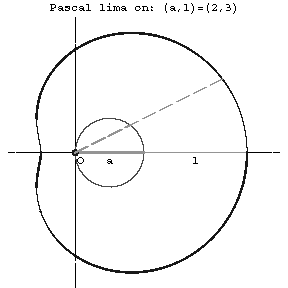Figure: p071770a

The equation in rectangular coordinates is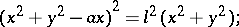in polar coordinates it is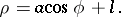The coordinate origin is a double point, which is an isolated point for, a node for, and a cusp for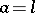(in this case Pascal's limaçon is a cardioid). The arc length can be expressed by an elliptic integral of the second kind. The area bounded by Pascal's limaçon isfor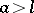the area of the inner leaf must be counted double in calculating according to this formula. The Pascal limaçon is a special case of a Descartes oval, it is an epitrochoid (see Trochoid).

The limaçon is named after E. Pascal, who first treated it in the first half of the 17th century.

How to Cite This Entry:
Pascal limaçon. Encyclopedia of Mathematics. URL: http://encyclopediaofmath.org/index.php?title=Pascal_lima%C3%A7on&oldid=12704
This article was adapted from an original article by D.D. Sokolov (originator), which appeared in Encyclopedia of Mathematics - ISBN 1402006098. See original article